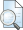## PENGARUH VARIASI PERENDAMAN TERHADAP NILAI STABILITAS MARSHALL PADA LASBUTAG CAMPURAN PANASPreview
Text

## Abstract

The road is a means of land transportation which consists of some materials, such as coarse aggregate, fine aggregate, filler (filler) and bitumen. In the process of maintenance, damage sometimes occur earlier than the service one of which is caused by the water factor. This study aimed to determine the effect of immersion (water) against the Marshall properties such as stability, flow on plastic, Results For Marshall, and air cavities. By using the same binder content (optimum), ie 2.5%, then the specimen is made of 3 x 9 specimens (for statistical tests), which is a cylinder with volume of 1200 grams of weight. The variation of immersion time used in this study were 2, 4, 6, 8, 10, 12, 24, 48, and 72 hours and for each - each variation of immersion time using 3 specimens. The results showed that there is the effect of immersion on the Stability of Marshall on the nature Lasbutag Mixed Heat, which is shown by the equation: Y = - 9.618x + 2098.2 (F-count regression = 7706> F-table = 4:24 at probability level 0.05). Marshall stability and is expected to reach below the requirements after the age of 140.18 hours. As for the plastic flow, Results For Marshall and air cavities less effect on the period of immersion. Where to values on plastic flow, with the equation: Y = 1982 + 0.0268X - 0.0005X2 + 4E-07 (F-count regression = 1105 <F-table = 3:01 at probability level 0.05). For the results shown for Marshall equation: Y = 16,744 - 0.4906X - 0.0058X2 (F-count regression = 1.65 <F-table for the level of probability = 5.61 0.01). And for the air cavity is indicated by the equation: Y = 9.8285 - 0.0162X (F-count regression = 1:21 <F-table = 4:24 at probability level 0.01).

Item Type: Thesis (Other) T Technology > TA Engineering (General). Civil engineering (General) Faculty of Engineering > Department of Civil Engineering (22201) Rayi Tegar Pamungkas 19 Jun 2012 08:51 19 Jun 2012 08:51 http://eprints.umm.ac.id/id/eprint/8603View Item